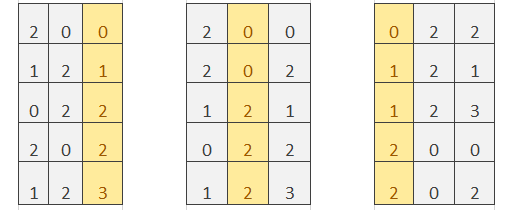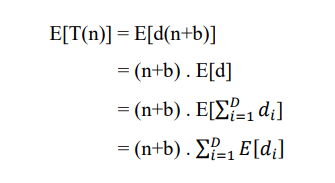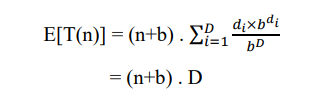×

Search anything:

# Time and Space complexity of Radix Sort

#### Time Complexity Algorithms Sorting AlgorithmsIndian Technical Authorship Contest starts on 1st July 2023. Stay tuned.

In this article, we have explained the Time and Space complexity of the Radix Sort with Mathematical and Intuitive Analysis.

2. Time Complexity analysis.
3. Worst case time complexity
4. Best case time complexity
5. Average case time complexity
6. Space Complexity analysis.
7. Conclusion on time and space complexity
8. Comparison with other sorting algorithms

In short:

• Worst case time complexity: O(logb(mx)(n+b)); Only one element which has significantly large number of digits
• Best case time complexity: All elements have the same number of digits
• Average case time complexity: O(d(n+b))
• Space Complexity: O(n+b)

Radix sort is a sorting technique that sorts the elements digit to digit based on radix. It works on integer numbers. To sort the elements of the string type, we can use their hash value. This sorting algorithm makes no comparison.

In this article, we will work for base b=10 for simplicity. For base b, number of digits in a number mx is d = $\lfloor log_b(mx)+1 \rfloor$

Given an array of n elements arr. Here, we have implemented two functions named countingSort and radixSort.

### Algorithm:

1. mx = arr
2. for i = 1 to n-1
if mx < arr[i]
mx = arr[i]
4. i = 1
5. q = mx/i
6. while q > 0
countingSort(arr, n, i)
i = i * 10
q = mx/i


In radixSort, at first we need to find out the largest value. In step 6, while loop iterates over all digits of the mx from the end and calls the countingSort function. While loop calls the function for d (number of digits in mx) times.

countingSort(arr, n, div)

1. let cnt[0...b] and tempArray[0...n] be new arrays
2. for i = 0 to 9
cnt[i] = 0
3. for i = 0 to n-1
cnt[(arr[i] / div) % 10] = cnt[(arr[i] / div) % 10] + 1
4. for i = 1 to 9
cnt[i] = cnt[i-1] + cnt[i]
5. for i = n-1 downto 0
tempArray[cnt[(arr[i] / div) %10]] = arr[i]
cnt[(arr[i] / div) %10] = cnt[(arr[i] / div) %10] - 1
6. for i = 0 to n-1
arr[i] = tempArray[i]


In countingSort, at first, we need to initialize the cnt array with 0. Then, we store the occurrence of each digit of input elements in the cnt array. Example:
Let a number 123 and we need to find the 1st number from the end of it. So, it should be 3 and we'll increase the cnt.

In step 4, we calculate the cumulative sum of the cnt array. Now, cnt[i] holds the number of digits less than or equal to digit i.

We can get the index of each element of the arr in the cnt array. In step 5, place the arr elements in the correct position of the tempArray. In step 6, copy the tempArray to the arr.

Example:

Given input list- 22, 123, 121, 200, 202## Time Complexity analysis

The time complexity of above algorithm:

• Step 1 takes O(1) time.
• In Step 2, the for loop is executed for n-1 times so it takes O(n) time.
• Step 4 and Step 5 take O(1) time.
• In Step 6, the while loop is executed for d times so it takes O(d) time.

For countingSort-

• In Step 2, the for loop is executed for b (base value) times so it takes O(b) time.
• In Step 3, the for loop is executed for n times so it takes O(n) time.
• In Step 4, the for loop is executed for b times so it takes O(b) time.
• In Step 5, the for loop is executed for n times so it takes O(n) time.
• In Step 6, the for loop is executed for n times so it takes O(n) time.

The time complexity of radix sort is O(d*(n+b)).

## Worst case time complexity

The worst case in radix sort occurs when all elements have the same number of digits except one element which has significantly large number of digits. If the number of digits in the largest element is equal to n, then the runtime becomes O(n2). The worst case running time of Counting sort is O(n+b). If b=O(n), then the worst case running time is O(n). Here,the countingSort function is called for d times, where d = $\lfloor log_b(mx)+1 \rfloor$.

Total worst case complexity of radix sort is O(logb(mx)(n+b)).

## Best case time complexity

The best case occurs when all elements have the same number of digits. The best case time complexity is O(d(n+b)). If b = O(n), then time complexity is O(dn).

## Average case time complexity

In the average case, we have considered the distribution of the number of digits. There are D passes and each digit can take on up to b possible values. Radix sort doesn't depend on the input sequence, so we may keep n as a constant.
The running time of radix sort is, T(n) = d(n+b). Taking expectations of both sides and using linearity of expectation,Numbers with di digits will be b^di - b^(di-1), where b is base. So, Contribution of each di will be di * b^di / b^D.

As di grows, the value of the term grows to D exponentially. The sum will be less than b * D which we can assume to be O(D) for simplicity.The average case time complexity of radix sort is O(D*(n+b)).

## Space Complexity

In this algorithm, we have two auxiliary arrays cnt of size b (base) and tempArray of size n (number of elements), and an input array arr of size n.

Space complexity: O(n+b)

The base of the radix sort doesn't depend upon the number of elements. In some cases, the base may be larger than the number of elements.

## Conclusion on time and space complexity

Time Complexity: O(d(n+b))
Space Complexity: O(n+b)

Radix sort becomes slow when the element size is large but the radix is small. We can't always use a large radix cause it requires large memory in counting sort. It is good to use the radix sort when d is small.

## Comparison with other sorting algorithms

### Comparison based sorting algorithm:

• The lower bound of the comparison based sorting algorithm is nlog2n. But when d is constant radix sort runs in linear time. In some cases, it performs better than quicksort and mergesort.
• When d gets high, the time complexity of the radix sort is worse than other linearithmic sorting algorithms like merge sort, quicksort, etc.
• Insertion sort average case time complexity is O(n^2), whereas radix sort has better time complexity than insertion sort.

### Non-Comparison based sorting algorithm:

Radix sort, counting sort & bucket sort use bucket for sorting. Radix sort needs a base number of buckets.

• In counting sort, we need a bucket for each unique value. So, the number of buckets is 0 to mx (value of largest element). In the counting sort, the larger the element, the larger the size of the auxiliary array. So the complexity becomes worse when the element becomes too large. On the other hand, space complexity of the radix sort is better than the counting sort.
• Bucket sort requires dynamic memory. Bucket sort worst case time complexity is O(n^2), whereas radix sort is O(d(n+b)). Radix sort is stable but bucket sort is stable if its sub-routine sort is stable.